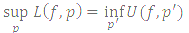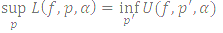# On the Review of Riemann and Riemann Stieljtes Integrals

## Abstract

This work seeks an understanding of integration as a generalization of the summation process either in the Riemann or the Riemann Stieljtes sense as the case may be.

First, on having an interval [a, b] in ℝ, a partition is constructed with which the Riemann sums R(f, p) is calculated and if such sums tends to a finite  limit and the mesh m(p) tends to zero then the function is intergrable and the Riemann integration of such function is defined asfor any partition p ∈ ℝ  and p' in [a, b] ∈ ℝ and  p' a refinement of  p

where U(f, p) and L(f, p)  have their usual meanings.

Again, With the interval [a, b] and a partition on it such that the Riemann Stieljtes sums of f  with respect to a, R (f, p, a) are calculated and if such sums tends to a finite limit as the mesh m(p) to zero then the function is Riemann Stieljtes integrable and such integral is then defined asfor any partitions p and p' in [a, b]  and p'  a refinement of p where  U(f, p', a) and L(f, p, a)have their usual meanings.

Further, we explored the various properties of the Riemann and Riemann Stieljtes integrals in form of theorems and lemma and in the last section we stated and proved some important and advanced results on the subject.

Keywords:
Intervals, partition, infimum, supremum, lower sum, upper sum, integral

## Article Details

How to Cite
Emmanuel, E. C. (2019). On the Review of Riemann and Riemann Stieljtes Integrals. Asian Research Journal of Current Science, 1(1), 45-57. Retrieved from http://globalpresshub.com/index.php/ARJOCS/article/view/794
Section
Review Article

## References

Chidume CE. Foundations of Riemans Inrtegration, (Monograph). The Abdusalam International Center for Theoritical Physics, Trieste. Italy; 1991.

Royden H. Real analysis. Macmillan New York; 1963.

Federer H. Geometric measure theory. Springer-Verlag, New York; 1969.

Stromberg K. An introduction to classical real analysis. Nadsworth Publishing Inc. Belmort; 1981.

Rudin W. Principles of mathematical analysis. 3rd Ed., McGraw Hill Book Company New York; 1976.

Trech WF. Introduction to real analysis. National Mathematical Centre Abuja Nigeria; 2010.

Krantz SG. The element of advanced mathematics. 2nd Ed., CRC Press, Boca Raton, FL; 2002.

Royden H. Real analysis. 3rd Ed., Stanford University, Preprintice Hall of India Private Limited New Delhi; 2008.